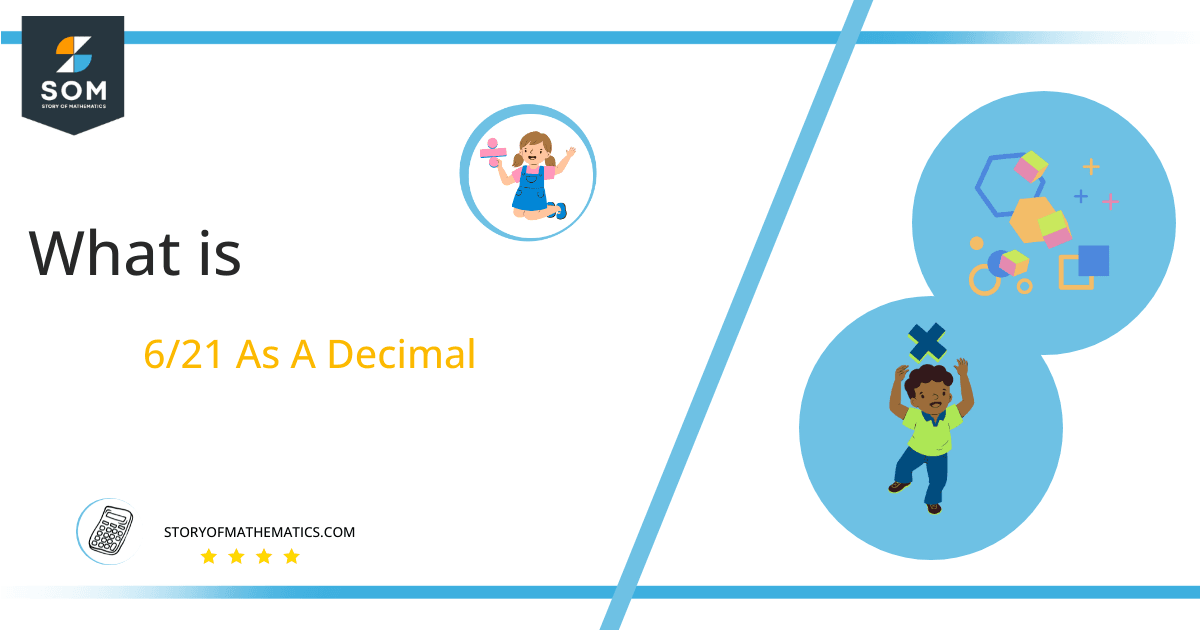# What Is 6/21 as a Decimal + Solution With Free Steps

The fraction 6/21 as a decimal is equal to 0.285.

A mathematical operation that permits you to solve complex and complicated problems related to division is called long division. Moreover, Long division is a method that breaks large numbers into manageable steps, thus, making a complex division much easier.

Here, we are more interested in the division types that result in a Decimal value, as this can be expressed as a Fraction. We see fractions as a way of showing two numbers having the operation of Division between them that result in a value that lies between two Integers.Now, we introduce the method used to solve said fraction to decimal conversion, called Long Division, which we will discuss in detail moving forward. So, let’s go through the Solution of fraction 6/21.

## Solution

First, we convert the fraction components, i.e., the numerator and the denominator, and transform them into the division constituents, i.e., the Dividend and the Divisor, respectively.This can be done as follows:

Dividend = 6

Divisor = 21

Now, we introduce the most important quantity in our division process: the Quotient. The value represents the Solution to our division and can be expressed as having the following relationship with the Division constituents:

Quotient = Dividend $\div$ Divisor = 6 $\div$ 21

This is when we go through the Long Division solution to our problem.

## 6/21 Long Division Method

We start solving a problem using the Long Division Method by first taking apart the division’s components and comparing them. As we have 6 and 21, we can see how 6 is Smaller than 21, and to solve this division, we require that 6 be Bigger than 21.

This is done by multiplying the dividend by 10 and checking whether it is bigger than the divisor or not. If so, we calculate the Multiple of the divisor closest to the dividend and subtract it from the Dividend. This produces the Remainder, which we then use as the dividend later.

Now, we begin solving for our dividend 6, which after getting multiplied by 10 becomes 60.

We take this 60 and divide it by 21; this can be done as follows:

60 $\div$ 21 $\approx$ 2

Where:

21 x 2 = 42

This will lead to the generation of a Remainder equal to 60 – 42 = 18. Now this means we have to repeat the process by Converting the 18 into 180 and solving for that:

180 $\div$ 21 $\approx$ 8

Where:

21 x 8 = 168

This, therefore, produces another Remainder which is equal to 180 – 168 = 12. Now we must solve this problem to Third Decimal Place for accuracy, so we repeat the process with dividend 120.

120 $\div$ 21 $\approx$ 5

Where:

21 x 5= 105

Finally, we have a Quotient generated after combining the three pieces of it as 0.285=z, with a Remainder equal to 15.Images/mathematical drawings are created with GeoGebra.